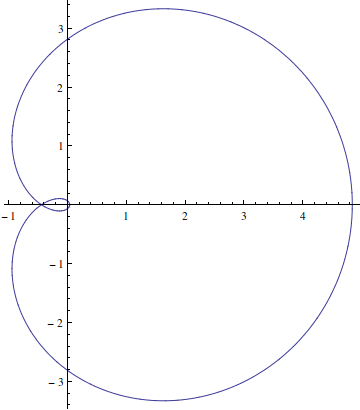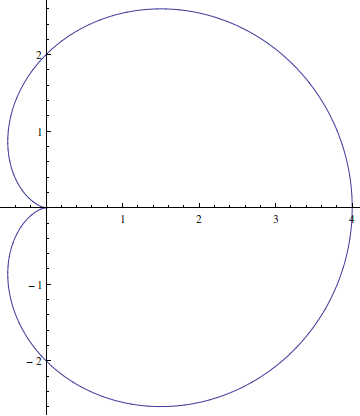Brian Bi
$\DeclareMathOperator{\ker}{ker} \DeclareMathOperator{\im}{im} \DeclareMathOperator{\diag}{diag} \DeclareMathOperator{\char}{char} \DeclareMathOperator{\lcm}{lcm} \newcommand\divides{\mathbin |} \newcommand\ndivides{\mathbin \nmid} \newcommand\d{\mathrm{d}} \newcommand\p{\partial} \newcommand\C{\mathbb{C}} \newcommand\N{\mathbb{N}} \newcommand\Q{\mathbb{Q}} \newcommand\R{\mathbb{R}} \newcommand\Z{\mathbb{Z}} \newcommand\pref{(\ref{#1})} \DeclareMathOperator{\Aut}{Aut} \DeclareMathOperator{\Gal}{Gal}$

Section 15.10. The Fundamental Theorem of Algebra

Exercise 15.10.1 Let $$\overline{\Q}$$ denote the algebraic numbers. Suppose $$f \in \overline{\Q}[x]$$. Then $$f$$ has a root $$\alpha \in \C$$. The extension $$\overline{\Q}(\alpha)/\overline{\Q}$$ is finite, therefore algebraic, and the extension $$\overline{\Q}/\Q$$ is also algebraic by definition of $$\overline{\Q}$$. We showed in Exercise 15.3.10 that algebraic extensions are transitive, so $$\overline{\Q}(\alpha)/\Q$$ is algebraic. Therefore there exists $$g \in \Q[x]$$ such that $$g(\alpha) = 0$$. But $$g$$ splits completely over $$\C$$ and its roots are algebraic numbers, so $$\alpha$$ is one of these, and is itself algebraic.

Exercise 15.10.2 Fix $$p$$. Let $$F_i$$ denote the finite field of order $$p^{i!}$$. By Theorem 15.7.3(e), each $$F_i$$ contains a copy of $$F_{i-1}$$. We can therefore form the ascending union $$F = \bigcup_{i=1}^\infty F_i$$, which itself has the structure of a field. If $$f \in \mathbb{F}_p[x]$$ is irreducible of degree $$d$$, then $$f$$ appears as a factor of $$x^{p^d} - x$$, and therefore has a root in $$\mathbb{F}_{p^d} \subseteq F_d \subseteq F$$.

We need to show that $$F$$ is algebraically closed. The argument is very similar to that used in the previous problem. Since each $$F_i$$ is a finite extension of $$\mathbb{F}_p$$, it is algebraic, and since each element of $$F$$ is an element of some $$F_i$$, $$F$$ is an algebraic extension of $$\mathbb{F}_p$$. Let $$g \in F[x]$$. Then $$g$$ has a root $$\alpha \in K$$ where $$K$$ is some extension of $$F$$. Since both $$F(\alpha)/F$$ and $$F/\mathbb{F}_p$$ are algebraic extensions, the extension $$F(\alpha)/\mathbb{F}_p$$ is also algebraic. This implies that there exists $$h \in \mathbb{F}_p[x]$$ such that $$h(\alpha) = 0$$. But $$h$$ splits completely over $$F$$, therefore $$h$$ splits completely over $$K$$ and all of its roots lie in $$F$$. This implies that $$\alpha \in F$$. So $$F$$ is algebraically closed.

Exercise 15.10.3 When $$r$$ corresponds to the magnitude of some root of $$f$$, the curve $$f(C_r)$$ will pass through the origin, so its winding number will be undefined. It is when $$f(C_r)$$ passes through such singular curves that the winding number can change discontinuously from just above $$r$$ to just below $$r$$.

For example, using Mathematica, we can plot the curves $$f(C_r)$$ where $$r = 1.2, 1.0, 0.8$$ respectively and $$f(z) = z^2 + 2z + 1$$:As $$r$$ decreases from above 1 to below 1, its winding number around the origin changes discontinuously from 2 to 1 as $$r$$ passes through 1, where the curve $$f(C_r)$$ passes through the origin. Moreover, a similar issue occurs with the total curvature, which may still be defined for curves that pass through the origin, but not for curves that exhibit a cusp, as the middle curve does above.